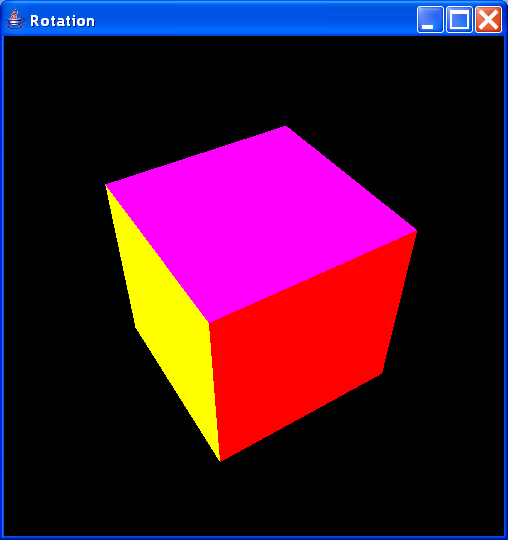# Simulate a matrix. Provides method to travers vectors that compose the matrix. : Matrix « 2D Graphics GUI « Java

Simulate a matrix. Provides method to travers vectors that compose the matrix.

```
/*
* Copyright  2009 Perseus Project - Tufts University <http://www.perseus.tufts.edu>
*
* This file is part of UtilPerseus.
*
* UtilPerseus is free software: you can redistribute it and/or modify
* the Free Software Foundation, either version 3 of the License, or
* (at your option) any later version.
* UtilPerseus is distributed in the hope that it will be useful,
* but WITHOUT ANY WARRANTY; without even the implied warranty of
* MERCHANTABILITY or FITNESS FOR A PARTICULAR PURPOSE.  See the
* GNU General Public License for more details.
* You should have received a copy of the GNU General Public License
* along with UtilPerseus.  If not, see <http://www.gnu.org/licenses/>.
*/

//package eu.himeros.util;

import java.util.Vector;

/**
* Simulate a matrix. Provides method to travers vectors that compose the
* matrix.
*
* @author federico
*/
public class Matrix {
private Vector<Integer>[] v = null;
private final int sizeDefault = 1000;
private int dim = 2;

/**
* Default constructor.
*/
public Matrix() {
init(dim, 1000);
}

/**
* Init the matrix with dimension.
*
* @param dim
*            dimension.
*/
public Matrix(int dim) {
this.dim = dim;
init(dim, 1000);
}

/**
* Constructor that init the matrix with number of dimensions and size.
*
* @param dim
*            dimension.
* @param size
*            size.
*/
public Matrix(int dim, int size) {
init(dim, sizeDefault);
}

/**
* Init the matrix with number of dimensions and size.
*
* @param dim
*            dimension.
* @param size
*            size.
*/
public void init(int dim, int size) {
v = new Vector[dim];
for (int i = 0; i < dim; i++) {
v[i] = new Vector<Integer>(size);
}
}

/**
* Add an array of integers, traversing all the vectors.
*
* @param x
*            the integer array to add.
*/
for (int i = 0; i < x.length; i++) {
}
}

/**
* Add integers to the first two vectors.
*
* @param x1
*            integer to add to the first vector.
* @param x2
*            integer to add to the second vector.
*/
public void add(int x1, int x2) {
// for bi-dimensional arrays
}

/**
* Return an int array, traversing all the vectors at the given index.
*
* @param idx
*            the index.
* @return the int array.
*/
public int[] get(int idx) {
int[] res = new int[dim];
for (int i = 0; i < dim; i++) {
res[i] = v[i].get(idx);
}
return res;
}

/**
* Remove an item in all the vectors at the given index.
*
* @param idx
*            the index.
*/
public void remove(int idx) {
for (int i = 0; i < dim; i++) {
v[i].remove(idx);
}
}

/**
* Get the vector array.
*
* @return the vector array.
*/
public Vector[] getVectorArray() {
return v;
}

/**
* Get size of the matrix.
*
* @return size.
*/
public int size() {
return v.size();
}

}

```

### Related examples in the same category

 1 Implementation of a 4x4 matrix suited for use in a 2D and 3D graphics rendering engine 2 Rotations in a three-dimensional space3 This class represents a lower (or upper) triangle matrix that stores ints. 4 The Java Matrix Class provides the fundamental operations of numerical linear algebra 5 Vector extends Matrix 6 A 3x3 matrix implementation 7 4 x 4 Matrix 8 Various geometric transformations on matrix form 9 Inertia Matrix